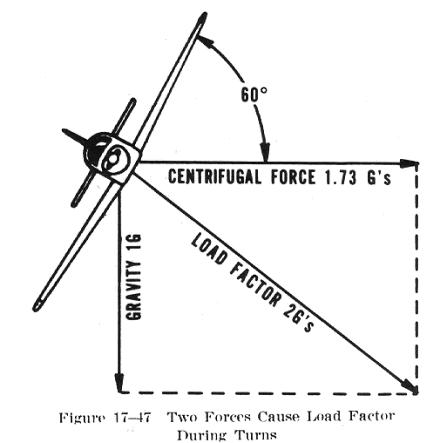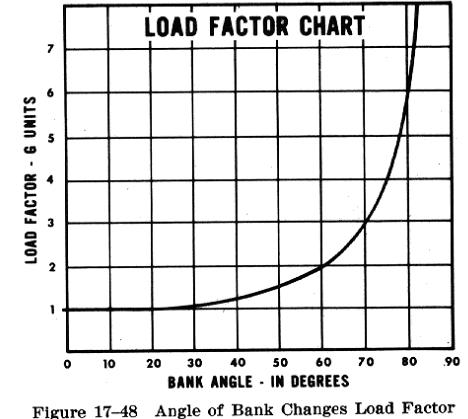In a constant altitude, coordinated turn in any airplane, the load factor is the result of two forces - centrifugal force and gravity (Fig. 17-47). For any given bank angle, the rate of turn varies with the airspeed; the higher the speed, the slower the rate of turn. This compensates for added centrifugal force, allowing the load factor to remain the same.Fig. 17-48 reveals an important fact about turns - that the load factor increases at a terrific rate after a bank has reached 45 or 50 degrees. The load factor for any airplane in a 60 degree bank is 2 G's. The load factor in an 80 degree bank is 5.76 G's. The wing must produce lift equal to these load factors if altitude is to be maintained.

It should be noted how rapidly the line denoting load factor rises as it approaches the 90 degree bank line, which it reaches only at infinity. The 90 degree banked, constant altitude turn mathematically is not possible. True, an airplane may be banked to 90 degrees but not in a coordinated turn; an airplane which can be held in a 90 degree banked slipping turn is capable of straight knife edged flight. At slightly more than 80 degrees the load factor exceeds the limit of 6 G's, the limit load factor of an acrobatic airplane.For a coordinated, constant altitude turn, the approximate maximum bank for the average general aviation airplane is 60 degrees. This bank and its resultant necessary power setting reach the limit of this type of airplane. An additional 10 degree bank will increase the load factor by approximately 1 G (Fig. 17-48), bringing it close to the yield point established for these airplanes.